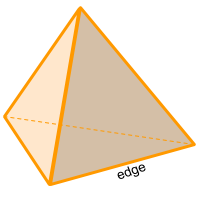Home » Polyhedra » Platonic Solids » Tetrahedron

# Volume of a TetrahedronVolume =
√2/12
× edge³

## Volume calculator for a tetrahedron

Enter the radius and the height of the tetrahedron to get the volume automatically.

## Description, how many faces, edges and vertices are there in a tetrahedron

The tetrahedron, is a triangular pyramid, where each face is an equilateral triangle; In case one of the faces (triangles) is not equal to the rest, it is considered an irregular tetrahedron or as a triangular pyramid and another formula is used to calculate the volume.

A tetrahedron (tetra = 4 and hedron = face), has 4 faces (equilateral triangles), has 6 edges, and has 4 vertices where 3 edges meet.

## Formula for the volume of a tetrahedron

To calculate the volume of a tetrahedron divide the square root of 2 into 12, then multiply the result by the edge to the power of 3 (since the tetrahedron is made up of equilateral triangles, you can use any edge). You can also use the online calculator to calculate the volume of the tetrahedron automatically.

Volume =
√2/12
× edge³

Share